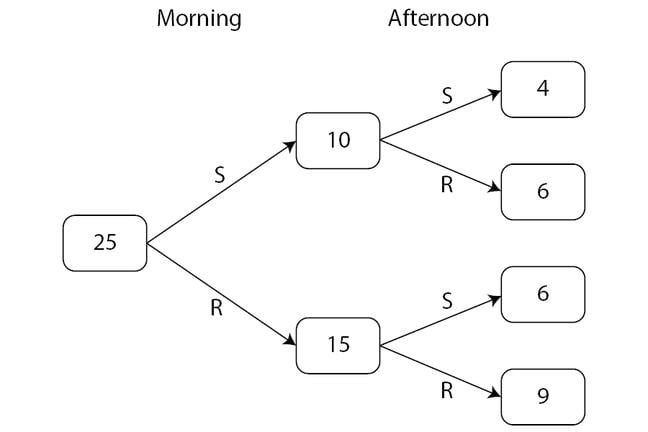## Want to keep learning?

This content is taken from the Cambridge University Press's online course, Teaching Probability. Join the course to learn more.
1.14

## Cambridge University PressExpected frequencies are our next step towards the probability concept.

# From empirical results to expected frequencies

So far, we have worked entirely with experimental data. We have deliberately used a mathematical model for this, as the difference between, say, a rainy morning and sunny afternoon, and a sunny morning and a rainy afternoon, is so much more obvious than between a Head and a Tail, and a Tail and a Head, on a coin. The spinner chosen also means we do not start from any assumptions about outcomes being equally likely - there is clearly more blue than yellow.

The next step is to look at the variation in results across the class. Through discussion, it should be possible to convince students that the mean results obtained by averaging the results of all the groups will help to smooth the data and reveal the long term behaviour of the system. (A spreadsheet simulation can also be used to achieve this.)

After this, we change the focus to looking at what we would expect the results to be. We ask students: ‘What percentage of the spinner is blue?’, and ask them to discuss and justify their suggestions. Following this discussion, we reveal that the spinner is actually 60% blue and 40% yellow.

We then start filling in the expected frequency diagram above:

• We start by writing the 25 into the first box, to represent the total number of days.
• Then we ask, ‘How many times would you expect it to be sunny in the morning, if the spinner is 40% yellow?’ [10 - i.e. 40% of 25]
• Next, we complete the ‘morning’ outcomes: ‘If 10 of the mornings were sunny, how many were rainy?’ [The remainder – i.e. 15]
• We compare these expected frequencies (10 and 15) to the class results - they should be close!
• Then we move on to the afternoon outcomes, making use of the narrative implied by the diagram: ‘So, 10 days with a sunny morning; how many of those would you expect to have a sunny afternoon? [4 – 40% of 10] And what can you say about the others? [The remaining 6 sunny mornings were followed by wet afternoons.]
• We complete the bottom branches (RS and RR) in a similar way.

With the diagram completed, we are on the way to understanding that the probability of a day being sunny throughout is 4/25.

As we build up the diagram, we make sure that students are aware of the difference between recording experimental data on a frequency tree and constructing the expected frequency tree. This is essentially the difference between observed relative frequency and theoretical probability.

Teachers sometimes tell us that their ‘standard’ approach would be to introduce and use probability much earlier in the teaching sequence. Why do you think we have chosen to do things this way?